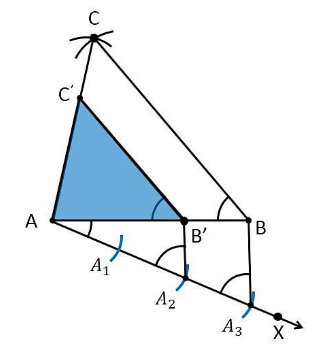# Construct a triangle of sides 4 cm, 5 cm and 6 cm and then a triangle similar to it whose sides are 2/3 of the corresponding sides of the first triangle. Give the justification of the construction

To construct a triangle from given conditions

1. Let us draw a line segment AB which measures 4 cm, i.e., AB = 4 cm.

2. On considering the point A as centre, and draw an arc of radius 5 cm.

3. Let us, take the point B as its centre, and draw an arc of radius 6 cm.

4. The arcs drawn will intersect each other at point C.

5. Now, we get AC = 5 cm and BC = 6 cm and therefore ΔABC is the required triangle.

6. Now drawa ray AX which makes an acute angle with the line segment AB on the opposite side of vertex C.

7. Position 3 points such as A1, A2, A3 (as 3 is greater between 2 and 3) on line AX such that it becomes AA1= A1A2 = A2A3.

8. Join the point BA3 and draw a line through A2which is parallel to the line BA3 that intersect AB at point B’.

9. Through the point B’, draw a line parallel to the line BC that intersect the line AC at C’.

10. Therefore, ΔAB’C’ is the required triangle.Justification

AB’ = (2/3)AB

B’C’ = (2/3)BC

AC’= (2/3)AC

From the construction, we get B’C’ || BC

∴ ∠AB’C’ = ∠ABC (Corresponding angles)

In ΔAB’C’ and ΔABC,

∠ABC = ∠AB’C From above)

∠BAC = ∠B’AC’ (Common)

∴ ΔAB’C’ ∼ ΔABC (From AA similarity criterion)

Therefore, AB’/AB = B’C’/BC= AC’/AC …. (1)

In ΔAAB’ and ΔAAB,

∠A2AB’ =∠A3AB (Common)

From the corresponding angles, we get,

∠AA2B’ =∠AA3B

Therefore, from the AA similarity criterion, we get

ΔAA2B’ and AA3B

So, AB’/AB = AA2/AA3

Therefore, AB’/AB = 2/3 ……. (2)

From the equations (1) and (2), we get

AB’/AB=B’C’/BC = AC’/ AC = 2/3

This can be expressed as

AB’ = (2/3)AB

B’C’ = (2/3)BC

AC’= (2/3)AC

Hence, justified.